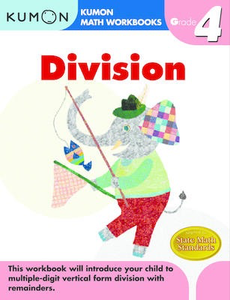# Grade 4 Division

• \$8.95

Division, Grade 4 is in the "Kumon Math Workbooks: Calculation Skills" series designed for grades 1-6. This workbook will introduce students to subtracting one-digit numbers from one-and-two digit numbers. Exercises include drill division problems as well as exercises where students fill in the missing numbers by using division patterns. Activities also emphasize addition, subtraction, multiplication & division review; vertical form division; five-digit by three-digit division; remainders; and the order of operations. Pages provide the number of points to assign per problem, and there's room at the top to write in how many problems students got right alongside their name and the date.